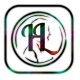# Logic Function and Boolean Algebra HSEB, NEB Old Questions# Logic Function and Boolean Algebra HSEB, NEB Old Questions

1. 2076: What is Boolean algebra? Describe AND and NAND gates with logic symbol, truth table and Venn diagram.[4+6]
2. 2075: Describe AND, OR, NOR gate with truth table, symbols and algebraic expressions.
3. 2075: Describe any 5 logic gates with truth table and gate symbol.
4. 2074: Describe AND, OR, NOR and XNOR gate with truth table, symbols and Venn diagram..
5. 2074: Describe AND, OR, NOR and NOT gate with truth table, symbols and Venn diagram.
6. 2074: Explain any 4 logic gates with truth table, symbol and Venn diagram.
7. 2073: What is logic gate? Describe AND, OR, NOR logic gate with truth table, gate symbol and Boolean expression.[2+8]
8. 2073: What is Boolean logic? Describe AND, OR, NOR logic gate with truth table and logic symbols.[2+8]
9. 2073:Describe Boolean algebra. Explain AND, OR, NAND and XOR gate with truth table and logic data.[2+8]
10. 2072, 2071,2070: Describe any 5 logic gates with truth table and gate symbol.
11. 2072: What is logic gate? Explain the types of gates with truth table.
12. 2071: Define logic gate. Explain the types of gates with truth table.[2+8]
13. 2071: Define logic gate. Explain the types of gates with truth table.[2+8]
14. 2070: Explain the types of gates with truth table.
15. 2070: Define logic gate. Explain the types of gates with truth table and gate symbol.[2+8]
16. 2069: What is Boolean algebra? Describe AND gate, OR gate, and Not Gate and NAND gate with gate symbol and truth table.[2+8]

1. 2076, 2073: Describe the De Morgan’s Law.
2. 2076: What is Associative law? Explain OR gate with truth table and logic symbol.
3. 2075: What is truth table? Explain AND gate with truth table and logic symbol.
4. 2074: Explain different types of bus architecture.
5. 2074: State and prove De Morgan’s theorem.
6. 2072: Give the truth table and logical symbols of AND, OR, and NOT gates of Boolean algebra.
7. 2069: Differentiate between NAND and NOR gate with truth table.
8. 2068: What is Boolean algebra? Differentiate between NAND and NOR gate with truth table.
9. 2067: What are the computer gates? Differentiate between NAND and NOR gate with truth table.
10. 2066: What are the logic gates? Explain the NAND gate with table.
11. 2065: What are logic gates? Differentiate between NAND and NOR gate with truth table.
12. 2064: Construct the truth table of XOR and XNOR operations of Boolean algebra.
13. 2063: What is NOR gate and construct its truth table.
14. Define a NOR gate and draw its logic symbol.
15. 2061: Construct truth table for NAND operation.
16. 2060: Write the truth table for NOR operation of Boolean algebra.
17. 2059: Write the truth table of NAND operation of Boolean algebra.
18. 2058: Construct the truth table of the AND and OR operation of Boolean algebra.
19. 2057: Define Boolean function. Construct truth table for AND operation of Boolean Algebra.

Write short notes on:

1. 2075: De Morgan’s Law